# Chaos in Low-Dimensional Lotka-Volterra Models of Competition

J. A. Vano, J. C. Wildenberg, M. B. Anderson, J. K Noel and J. C. Sprott

Received 5 September 2005, in final form 18 August 2006
Published 15 September 2006

### ABSTRACT

The occurrence of chaos in basic Lotka-Volterra models of four competing species is studied. A brute-force numerical search conditioned on the largest Lyapunov exponent (LE) indicates that chaos occurs in a narrow region of parameter space but is robust to perturbations. The dynamics of the attractor for a maximally chaotic case are studied using symbolic dynamics, and the question of self-organized critical behaviour (scale-invariance) of the solution is considered.

Ref: J. A. Vano, J. C. Wildenberg, M. B. Anderson, J. K. Noel, and J. C. Sprott, Nonlinearity 19, 2391-2404 (2006)

The complete paper is available in PDF format.

Figure 1. Carrying simplex for equation (1) with parameters in equation (3).Figure 2. The dynamics on the boundary of the carrying simplex in figure 1. The tetrahedron has been unfolded and laid flat for better viewing.Figure 3. Attractor projected onto x1x2x3 space.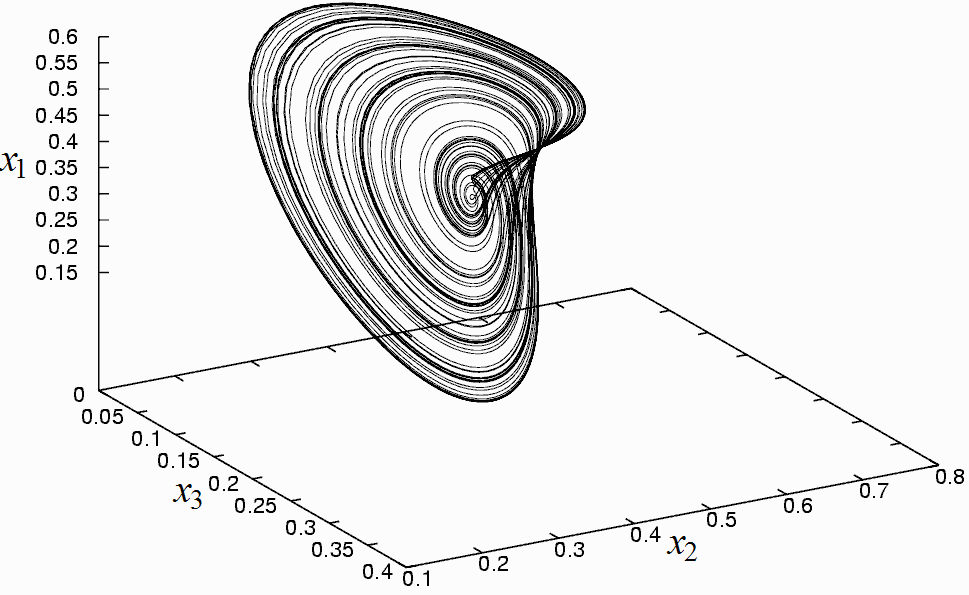Figure 4. Time series for each species (vertical scale is 0 to 1).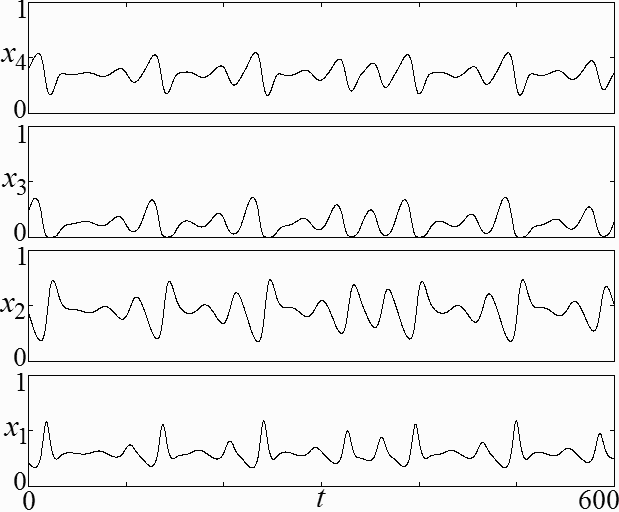Figure 5. Homoclinic connection projected onto the x1x2 plane.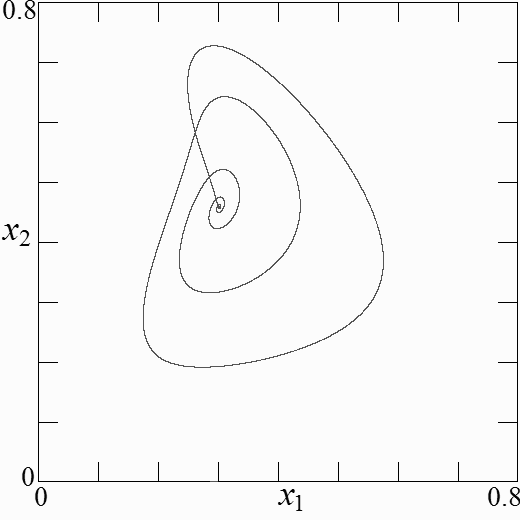Figure 6. (a) Bifurcation diagram showing successive maxima of x1 as the coupling variable s is increased and (b) the corresponding largest LE.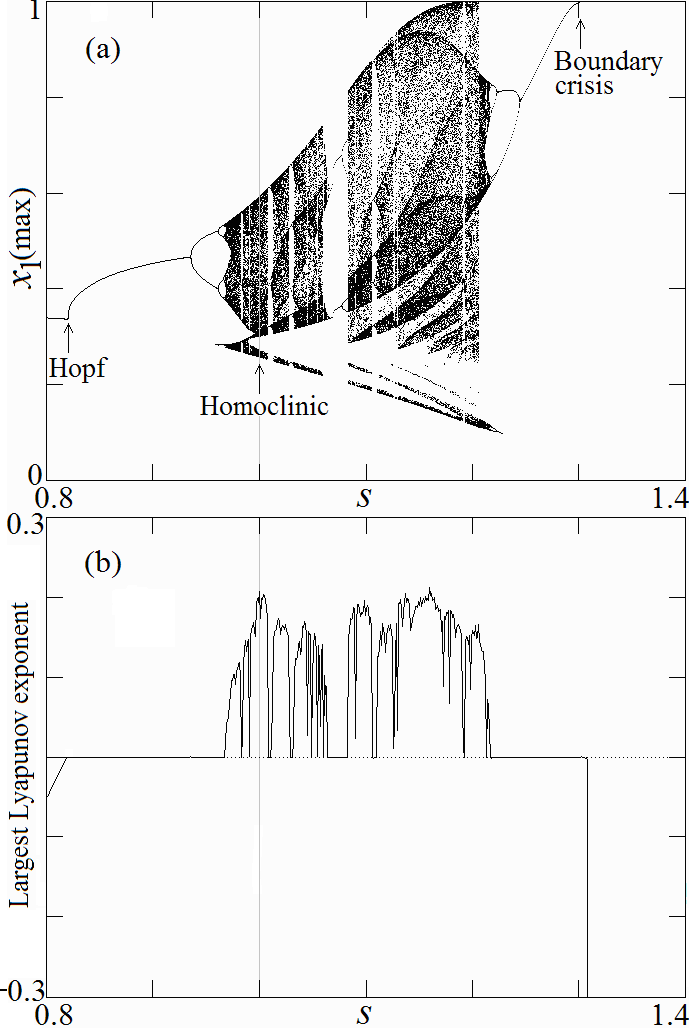Figure 7. This Monte Carlo scan over the space of initial conditions attempted to locate coexisting attractors in the range 0.8 < s < 1.4. The average variance of each variable was calculated along every orbit and summed. Significant differences in the variances for a single value of the bifurcation parameter s indicated multiple attractors. The fixed point Q124 is stable in the window s = 1.04 to s = 1.12, while Q34 becomes stable at s = 1.08 and is the only attractor for 1.31 < s < 1.4. Hysteresis occurs as the orbit remains at Q34 even if s is lowered below 1.31 until Q34 becomes unstable. At s = 1.06875 there are coexisting limit cycles and at s = 1.2375 the strange attractor coexists with a limit cycle, indicated by the single points (*).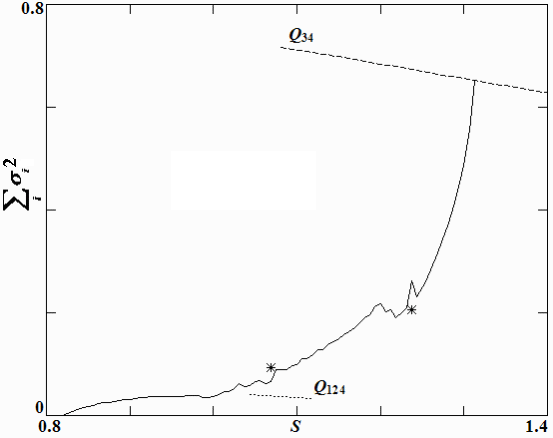Figure 8. Graph of symbolic dynamics of the attractor. The observed probabilities of the transitions are also indicated.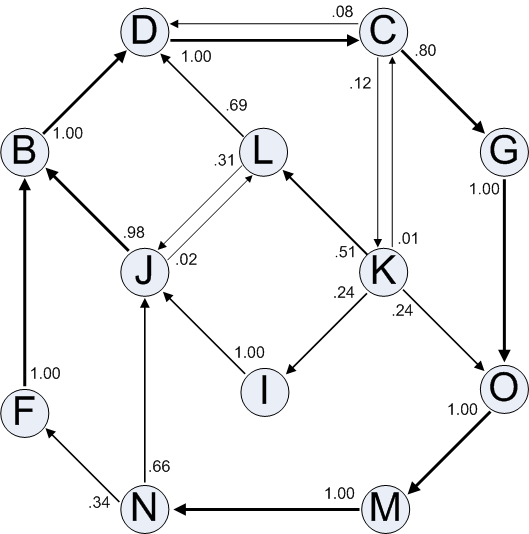Figure 9. The plot of Lambdan showing convergence to 0.0178, a close approximation to the ASE for the symbolic dynamics.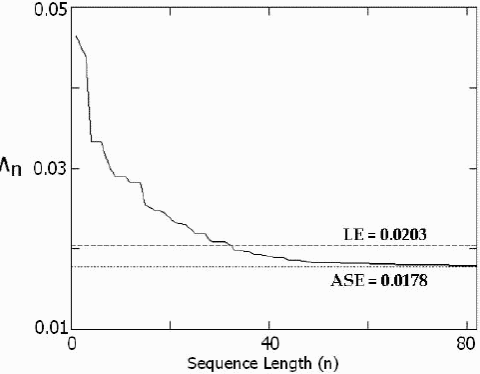Figure 10. Probability distribution function of volatility showing a power-law scaling (arbitrary scales).Figure 11. Probability of the largest LE, showing the rarity of chaos.